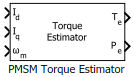# PMSM Torque Estimator

Estimate electromechanical torque and power

• Library:
• Motor Control Blockset / Controls / Control Reference

•## Description

The PMSM Torque Estimator block generates electromechanical torque and power estimates to enable field-oriented control of Permanent Magnet Synchronous Motor (PMSM). The block outputs the mathematically computed electromechanical torque for constant motor parameters. To measure an accurate torque value, we recommend that you use a physical sensor.

The block accepts feedback values of d- and q-axis current and mechanical speed as inputs.

### Equations

If you select `Per-Unit (PU)` in the Input units parameter, the block converts the inputs to SI units before performing any computation. After calculating the output, the block converts the output back to per unit values.

These equations describe the computation of electromechanical torque and power estimates by the block.

`${T}_{e}=\frac{3}{2}p\left\{{\lambda }_{m}{I}_{q}+\left({L}_{d}-{L}_{q}\right){I}_{d}{I}_{q}\right\}$`
`${P}_{\text{e}}={T}_{e}\cdot {\omega }_{\text{m}}$`

For detailed set of equations and assumptions that Motor Control Blockset™ uses for a PMSM, see Mathematical Model of PMSM.

where:

• ${L}_{\text{d}}$ and ${L}_{\text{q}}$ are the d-axis and q-axis stator winding inductances (Henry).

• ${I}_{\text{d}}$ and ${I}_{\text{q}}$ are the d-axis and q-axis current (Amperes).

• ${\lambda }_{\text{m}}$ is the permanent magnet flux linkage (Weber).

• $p$ is the number of pole pairs available in the motor.

• ${\omega }_{m}$ is the mechanical speed of the rotor (Radians/ sec).

## Ports

### Input

expand all

Current along the d-axis of the rotating dq reference frame.

Data Types: `single` | `double` | `fixed point`

Current along the q-axis of the rotating dq reference frame.

Data Types: `single` | `double` | `fixed point`

Mechanical speed of the rotor.

Data Types: `single` | `double` | `fixed point`

### Output

expand all

Electromechanical torque of the rotor.

Data Types: `single` | `double` | `fixed point`

Electromechanical power of the rotor.

Data Types: `single` | `double` | `fixed point`

## Parameters

expand all

Number of pole pairs available in the motor.

Stator winding inductance (henry) along the direct-axis of the rotating dq reference frame.

Stator winding inductance (henry) along the quadrature-axis of the rotating dq reference frame.

Peak permanent magnet flux linkage (weber).

Unit of the input values.

Base voltage (in Volts) for per-unit system.

#### Dependencies

To enable this parameter, set Input units to `Per-Unit (PU)`.

Base current (in Amperes) for per-unit system.

#### Dependencies

To enable this parameter, set Input units to `Per-Unit (PU)`.

Base speed (in rpm) for per-unit system.

#### Dependencies

To enable this parameter, set Input units to `Per-Unit (PU)`.

Base torque (in Nm) for per-unit system. See Per-Unit System page for more details.

This parameter is not configurable and uses a value that is internally computed using other parameters.

#### Dependencies

To display this parameter, set Input units to `Per-Unit (PU)`.

Base power (in W) for per-unit system. See Per-Unit System page for more details.

This parameter is not configurable and uses a value that is internally computed using other parameters.

#### Dependencies

To display this parameter, set Input units to `Per-Unit (PU)`.